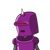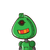# 10. Find the 5 rational numbers between the following 34 and 4/5​

10. Find the 5 rational numbers between the following 34 and 4/5​

### 2 thoughts on “10. Find the 5 rational numbers between the following 34 and 4/5​”

1.What are the five rational number between 3 /5 and 4/5?

Answer: 19/30, 20/30, 21/30, 22/30, 23/30.

In mathematics, a rational number is a type of real numbers. It can be defined as any number that can be expressed in the p/q form where q ≠ 0.

We can also say that any fraction falls under the class of rational numbers, where the denominator and numerator are integers and the denominator is not equal to zero. When the rational number that is a fraction is divided, the result will be in the form of decimal, that may be either repeating decimal or terminating decimal.

Properties of a rational number

The rational number is a subset of the real number, and all the properties of the real number system are obeyed by the rational number. A few of the rational number’s important properties are

If we multiply, add or subtract any two rational numbers, the outcome is always a rational number.

A rational number remains the same if both the numerator and denominator are divided or multiplied by the same factor.

If we add a rational number to zero, then we obtain the same number again.

Rational numbers are closed under subtraction, addition and multiplication.

To find five rational numbers between 3/5 and 4/5.

Multiply both the fraction by 6, we get

3×65×6

= 18/30

4×65×6

= 24/30

The fractions between 18/30 and 24/30 are

19/30, 20/30, 21/30, 22/30, 23/30.

2.Step-by-step explanation:

19/30,20/30,21/30,22/30,23/30